# RD Sharma Solutions for Class 9 Maths Chapter 16 Circles Exercise 16.3

The RD Sharma Class 9 solutions for the chapter “Circle” exercise 16.3 is given here. This study material specially designed by the experts at BYJU’S to help the students practice more effectively. In this exercise, students will learn about some important results on equal chords. These results are helpful to solve various circle problems. Students can click on below link and get RD Sharma class 9 Solution pdf now.

## Download PDF of RD Sharma Solutions for Class 9 Maths Chapter 16 Circles Exercise 16.3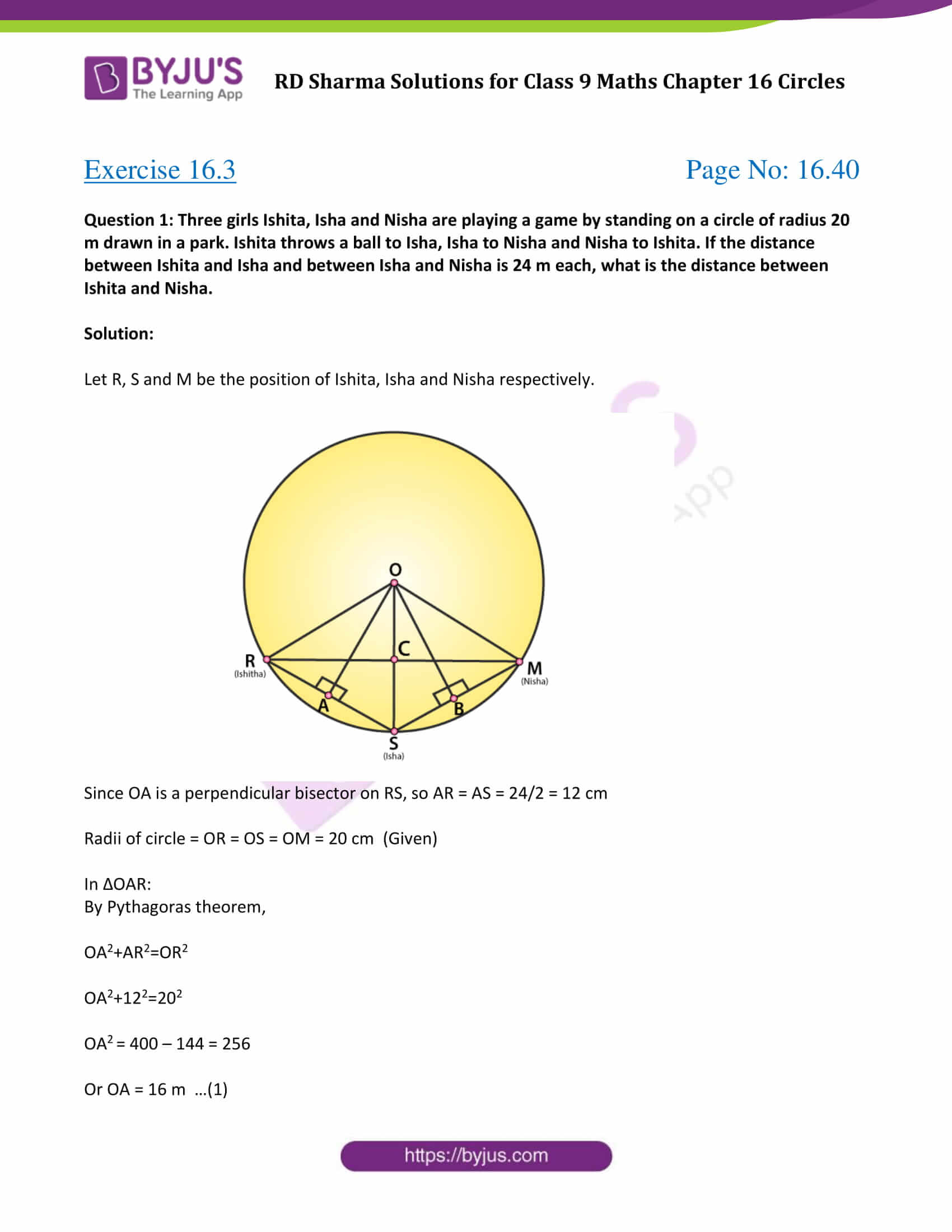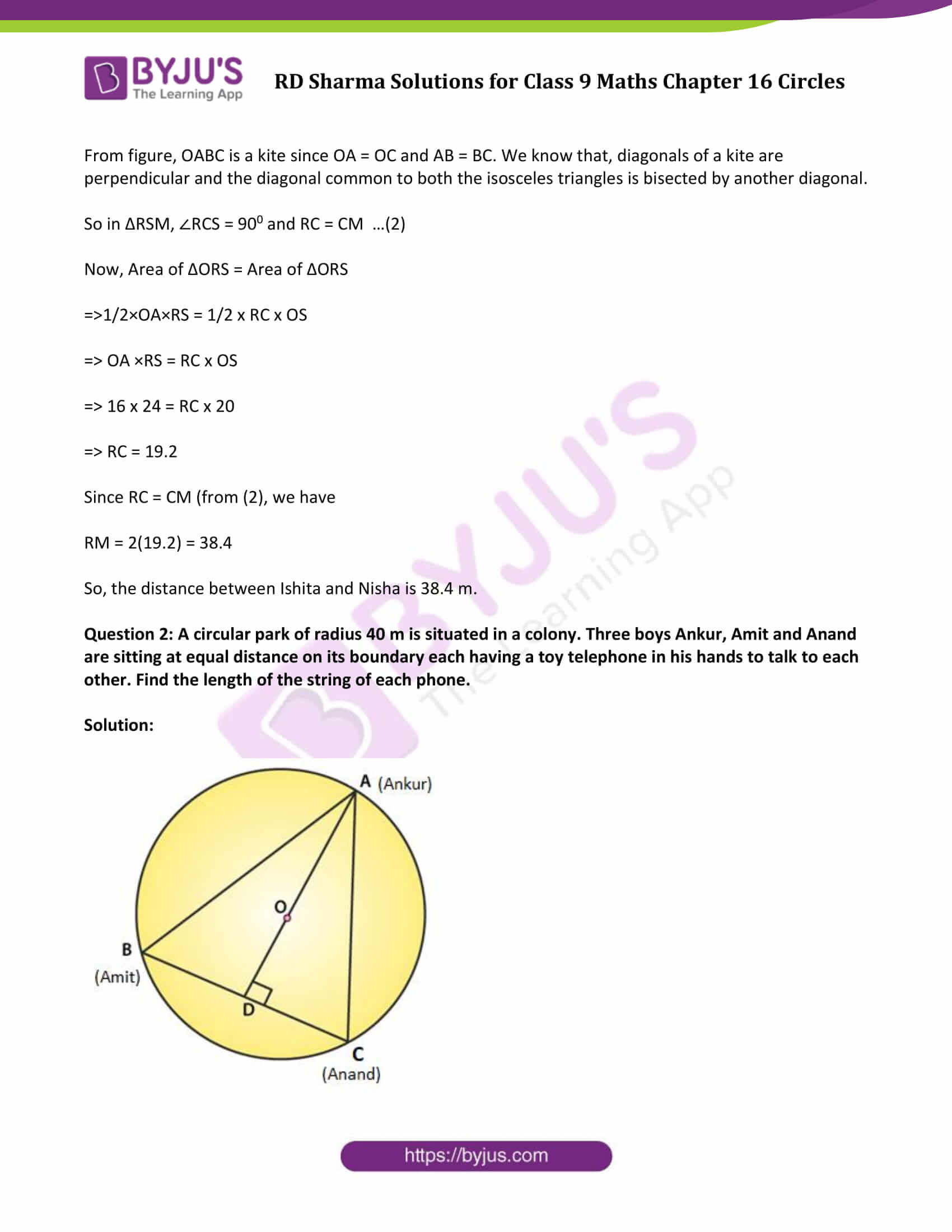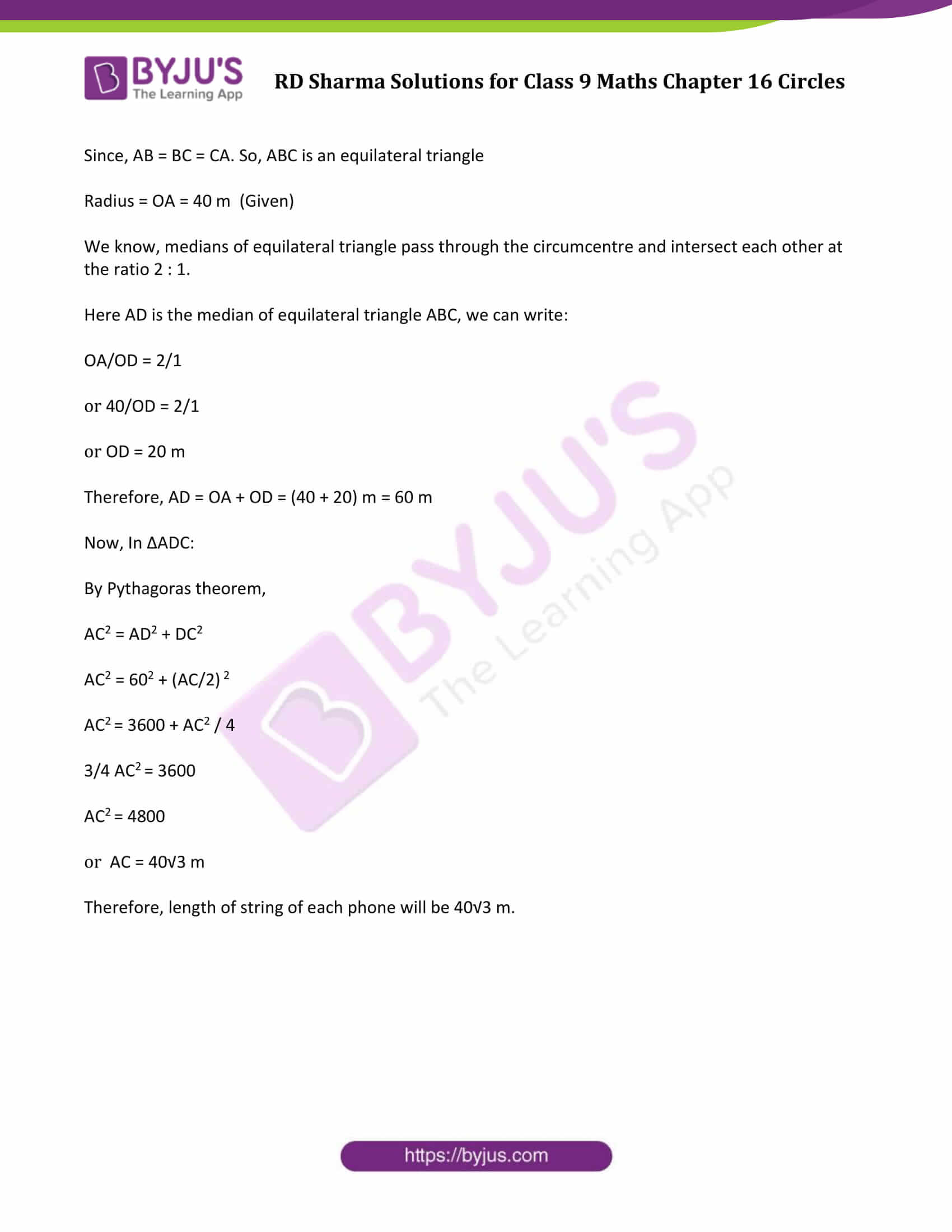### Access Answers to Maths RD Sharma Solutions for Class 9 Chapter 16 Circles Exercise 16.3 Page number 16.40

Question 1: Three girls Ishita, Isha and Nisha are playing a game by standing on a circle of radius 20 m drawn in a park. Ishita throws a ball to Isha, Isha to Nisha and Nisha to Ishita. If the distance between Ishita and Isha and between Isha and Nisha is 24 m each, what is the distance between Ishita and Nisha.

Solution:

Let R, S and M be the position of Ishita, Isha and Nisha respectively.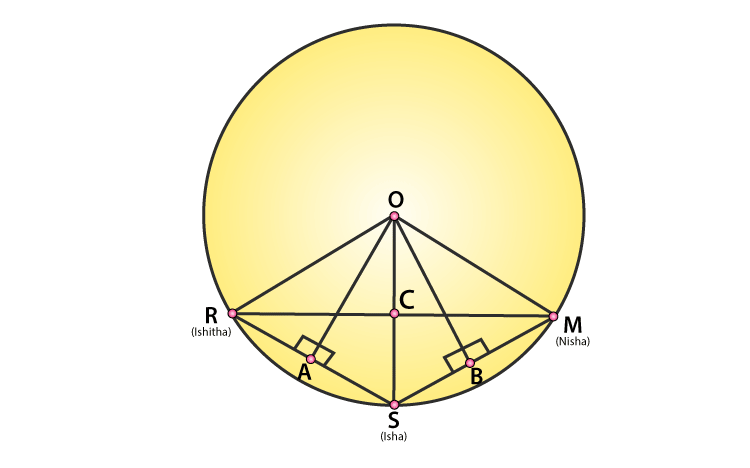Since OA is a perpendicular bisector on RS, so AR = AS = 24/2 = 12 cm

Radii of circle = OR = OS = OM = 20 cm (Given)

In ΔOAR:

By Pythagoras theorem,

OA2+AR2=OR2

OA2+122=202

OA2 = 400 – 144 = 256

Or OA = 16 m …(1)

From figure, OABC is a kite since OA = OC and AB = BC. We know that, diagonals of a kite are perpendicular and the diagonal common to both the isosceles triangles is bisected by another diagonal.

So in ΔRSM, ∠RCS = 900 and RC = CM …(2)

Now, Area of ΔORS = Area of ΔORS

=>1/2×OA×RS = 1/2 x RC x OS

=> OA ×RS = RC x OS

=> 16 x 24 = RC x 20

=> RC = 19.2

Since RC = CM (from (2), we have

RM = 2(19.2) = 38.4

So, the distance between Ishita and Nisha is 38.4 m.

Question 2: A circular park of radius 40 m is situated in a colony. Three boys Ankur, Amit and Anand are sitting at equal distance on its boundary each having a toy telephone in his hands to talk to each other. Find the length of the string of each phone.

Solution: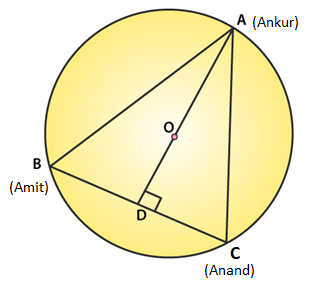Since, AB = BC = CA. So, ABC is an equilateral triangle

Radius = OA = 40 m (Given)

We know, medians of equilateral triangle pass through the circumcentre and intersect each other at the ratio 2 : 1.

Here AD is the median of equilateral triangle ABC, we can write:

OA/OD = 2/1

or 40/OD = 2/1

or OD = 20 m

Therefore, AD = OA + OD = (40 + 20) m = 60 m

By Pythagoras theorem,

AC2 = 602 + (AC/2) 2

AC2 = 3600 + AC2 / 4

3/4 AC2 = 3600

AC2 = 4800

or AC = 40√3 m

Therefore, length of string of each phone will be 40√3 m.

## RD Sharma Solutions for Class 9 Maths Chapter 16 Circles Exercise 16.3

Class 9 Maths Chapter 16 Circles Exercise 16.3 is based on the topic – Some important results on equal chords. Students can learn more Maths concepts using RD Sharma solutions and score good marks in examinations.